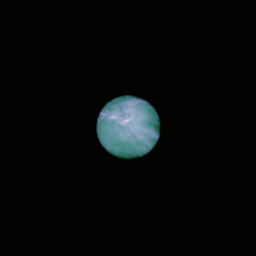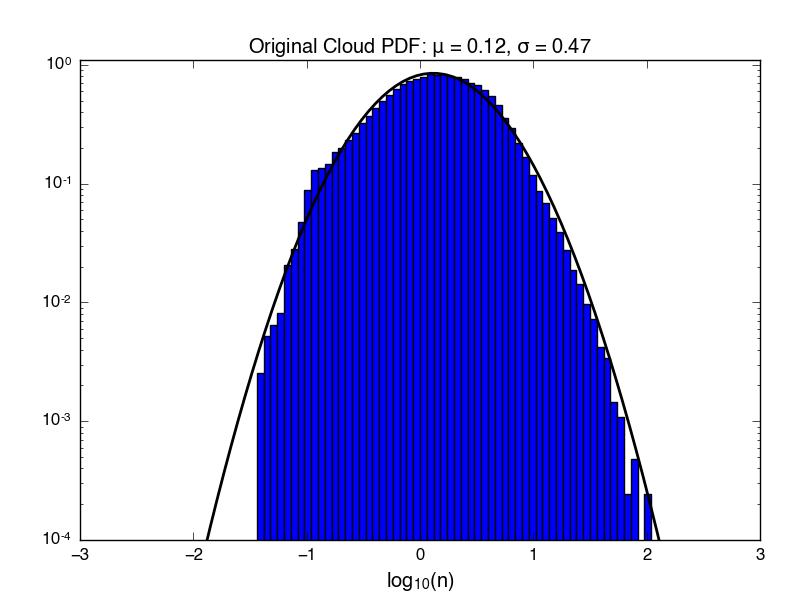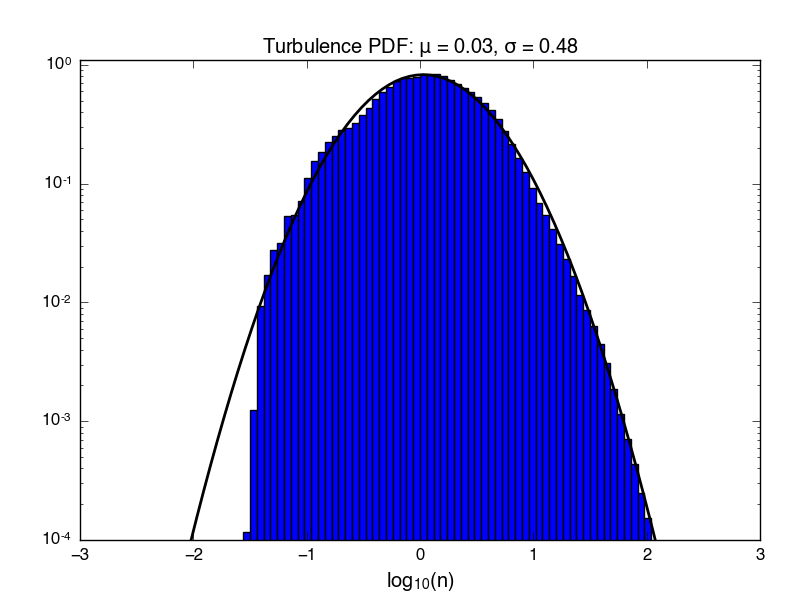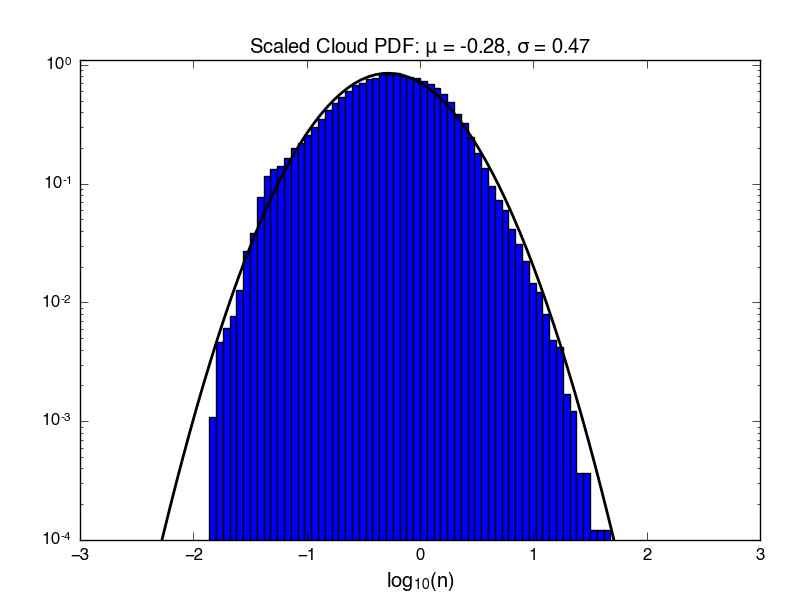Cloud PDFs

April 13, 2016

To create the initial conditions for the cloud-wind simulations, I need to find a way of scaling the raw data from the Mach 5 isothermal turbulence simulation data. Below are a png and pdf showing an excised region from the unscaled (but smoothed) data.I’m only plotting and fitting to the cloud data (not the wind), and I’ve not tapered the edge of the cloud, because I want statistics for the underlying distribution. The fitting parameters are the mean and standard deviation for a gaussian. As expected for isothermal supersonic turbulence, a log-normal distribution fits the data well.

We can compare the statistics for this region to a larger region of the turbulence simulation from which it is excised. Below is a pdf created using the $256^3$ region of the turbulence simulation that the cloud is taken from, without smoothing (the entire turbulence simulation was $1024^3$). Comparing the two, one can see that we have chosen a slightly overdense region for the cloud ICs.We’d like to match the cloud ICs to the sphere ICs as best we can, in terms of mean density and (if possible) cloud mass. What happens if I apply a linear scaling to the cloud density distribution shown in the first figure? Below is a PDF created by multiplying the original turbulence data by a constant factor (in this case 0.4), in order to match the mean density and initial mass of the $n_h = 1$ sphere simulation.The mean of the distribution has shifted, but the width stays the same.